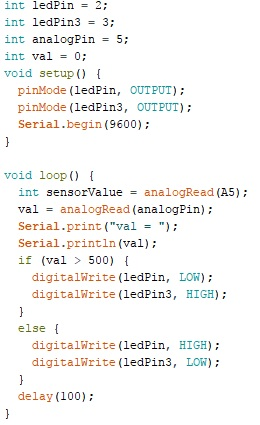# How to convert 0-1024 value from resistive soil moisture sensor to ohms?

How to convert 0-1024 value from resistive soil moisture sensor to ohms?
I connected the Arduino UNO R3 to the resistive soil moisture sensor and 220 ohms resistors. And this is my circuit diagram.

Code :coach_komkrit:
How to convert 0-1023 value from resistive soil moisture sensor to ohms?

provided you know the sensor characteristics (which you have NOT shared btw) and its linear, you can simply use the map function

``````map(sensorValue, 0, 1023, SensorMinOhm, SensorMaxOhm);
/*
where:
*/
``````

hope that helps....

Thank you very much.

It's common for people to want to convert the value read from analogRead into some units - voltage is common. It's not always necessary though and I doubt it is here.

With a soil moisture sensor, usually you're checking to see if it's time to water a plant or perhaps skip running lawn sprinklers. In that case, you can just figure out what the thresholds are in terms of what you get from the ADC. Store those values in constants and use them in your checks agains the raw value you read.

I don't know which type of sensor you are using.

Often resistive moisture sensors degrade very rapidly and poison you plants by the dissolved copper.

You could use small stainless steel rods for the probes.

Whandall:
I don't know which type of sensor you are using.

Often resistive moisture sensors degrade very rapidly and poison you plants by the dissolved copper.

You could use small stainless steel rods for the probes.

Resistive Soil Moisture Sensor, Icstation Humidity Detector, with Corrosion Resistant Probe, Digital Analog Signal Output for Arduino Garden Automatic Watering System (Pack of 2)
Amazon.com

You source it with a constant current source or sink, not a resistor to VCC or ground. When you have that then using ohm's law you can calculate the resistance. Accuracy of the current source and the AREF will affect these readings.

gilshultz:
You source it with a constant current source or sink, not a resistor to VCC or ground. When you have that then using ohm's law you can calculate the resistance. Accuracy of the current source and the AREF will affect these readings.

Thank you very much.

If the sensor is a voltage divider, using a known resistance R to Vcc and the soil resistance to ground,
then the formula for the soil resistance is
R (a / (1024-a)) [ where a is the analogRead value ].
This is the classic voltage divider formula. In C you'd need to force floating point calculation
for the divide, so you'd use

``````  a = analogRead (...) ;
soil_resistance = R * (a / (1024.0 - a)) ; // use float constant to force floating point divide
``````

There's no need to use a current source to measure this resistance, but you will need to find out the
value of R if this is indeed a resistive divider sensor - this calibration can be done by using a fixed
resistor in place of the soil and back-calculating from the formula.

MarkT:
If the sensor is a voltage divider, using a known resistance R to Vcc and the soil resistance to ground,
then the formula for the soil resistance is
R (a / (1024-a)) [ where a is the analogRead value ].
This is the classic voltage divider formula. In C you'd need to force floating point calculation
for the divide, so you'd use

``````  a = analogRead (...) ;
``````

soil_resistance = R * (a / (1024.0 - a)) ; // use float constant to force floating point divide

``````

There's no need to use a current source to measure this resistance, but you will need to find out the
value of R if this is indeed a resistive divider sensor - this calibration can be done by using a fixed
resistor in place of the soil and back-calculating from the formula.
``````

thank you very much.

This topic was automatically closed 120 days after the last reply. New replies are no longer allowed.